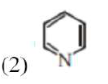# Choose the CORRECT answer from the options given below:

Question:

Statement I : Sodium hydride can be used as an oxidising agent.

Statement II : The lone pair of electrons on nitrogen in pyridine makes it basic.

Choose the CORRECT answer from the options given below:

1. Both statement I and statement II are false

2. Statement $I$ is true but statement II is false

3. Statement $\mathrm{I}$ is false but statement $\mathrm{II}$ is true

4. Both statement I and statement II are true

Correct Option: , 3

Solution:

(1) $\mathrm{NaH}$ (sodium Hydride) is used as a reducing reagent.In pyridine, due to free electron on $\mathrm{N}$ atom, it is basic in nature.

Hence statement $\mathrm{I}$ is false $\backslash$ II is true.